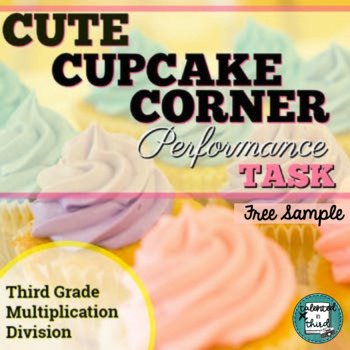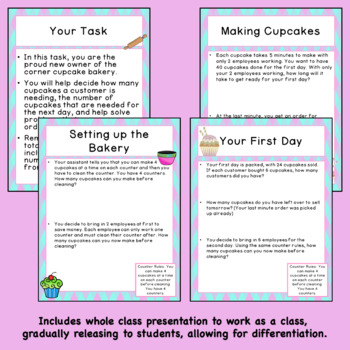# Multiplication and Division Word Problems - Grade 3 Cupcakes3rd - 4th
Subjects
Standards
Resource Type
Formats Included
• Zip
•Google Apps™
Pages
7 pagesThe Teacher-Author indicated this resource includes assets from Google Workspace (e.g. docs, slides, etc.).

#### Also included in

1. Do you need math enrichment activities for third grade? These SBAC style Performance Tasks are perfect for end of the year testing! These 9 Performance Tasks cover EVERY SINGLE math Common Core Standard!This Bundle of 9 SBAC Math Performance Tasks: Is great for prepping for Smarter Balanced Testing
\$28.00
\$31.50
Save \$3.50

### Description

Do you have students who don't understand multiplication and division? These Math Performance Task sample questions are taken from my full Cupcake Multiplication Performance Task based on SBAC performance tasks and focus on multiplication, division, time, subtraction, and more, but with a cupcake theme in which they are asked to run a bakery. This is a fun way to get students thinking critically about the skills they have learned. It is also a great way to practice and take some of the stress away from high stakes testing at the end of the year.

These tasks require students to complete a series of multi-step (2-3 step) word problems, modeled after the extensive performance tasks required by many of the states' testing, such as the Smarter Balanced Assessment.

Includes:

• Presentation for Whole Group Activity (could be more of a gradual release model)

Or buy the bundle and save \$\$\$\$\$

Total Pages
7 pages
N/A
Teaching Duration
N/A
Report this Resource to TpT
Reported resources will be reviewed by our team. Report this resource to let us know if this resource violates TpT’s content guidelines.

### Standards

to see state-specific standards (only available in the US).
Determine the unknown whole number in a multiplication or division equation relating three whole numbers. For example, determine the unknown number that makes the equation true in each of the equations 8 × ? = 48, 5 = __ ÷ 3, 6 × 6 = ?.
Use multiplication and division within 100 to solve word problems in situations involving equal groups, arrays, and measurement quantities, e.g., by using drawings and equations with a symbol for the unknown number to represent the problem.
Interpret whole-number quotients of whole numbers, e.g., interpret 56 ÷ 8 as the number of objects in each share when 56 objects are partitioned equally into 8 shares, or as a number of shares when 56 objects are partitioned into equal shares of 8 objects each. For example, describe a context in which a number of shares or a number of groups can be expressed as 56 ÷ 8.
Interpret products of whole numbers, e.g., interpret 5 × 7 as the total number of objects in 5 groups of 7 objects each. For example, describe a context in which a total number of objects can be expressed as 5 × 7.
Multiply one-digit whole numbers by multiples of 10 in the range 10–90 (e.g., 9 × 80, 5 × 60) using strategies based on place value and properties of operations.